# RD Sharma Solutions for Class 8 Maths Chapter 10 Direct and Inverse Variations Exercise 10.1

In Exercise 10.1 of Chapter 10, we shall deal with problems based on direct variations (if two quantities are linked in such a way that if one quantity increases, the corresponding quantity also increases and vice-versa, such a variation is called direct variation). Tutors at BYJU’S have designed the solutions for RD Sharma Solutions Maths. For students who wish to achieve good marks in their exams and build their confidence level, practising RD Sharma Class 8 textbook is essential. Students can download the pdf from the links provided below.

## Download the pdf of RD Sharma Solutions for Class 8 Maths Exercise 10.1 Chapter 10 Direct and Inverse Variations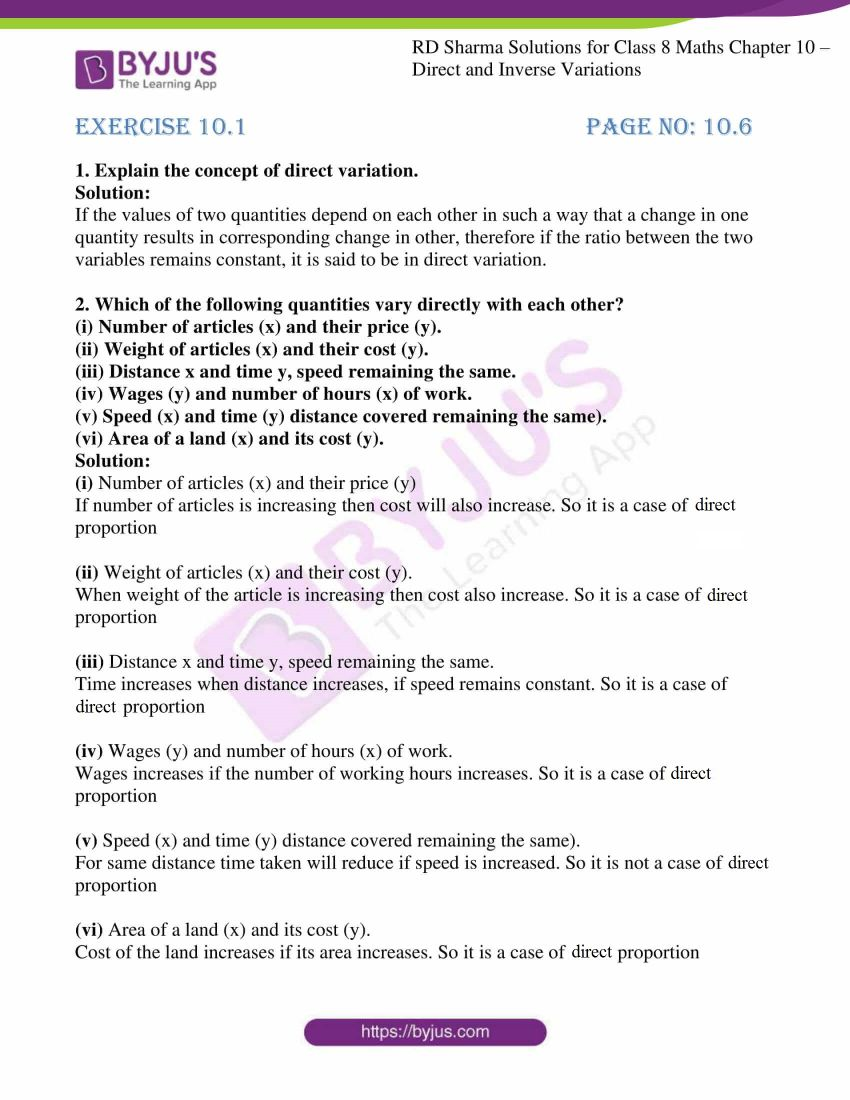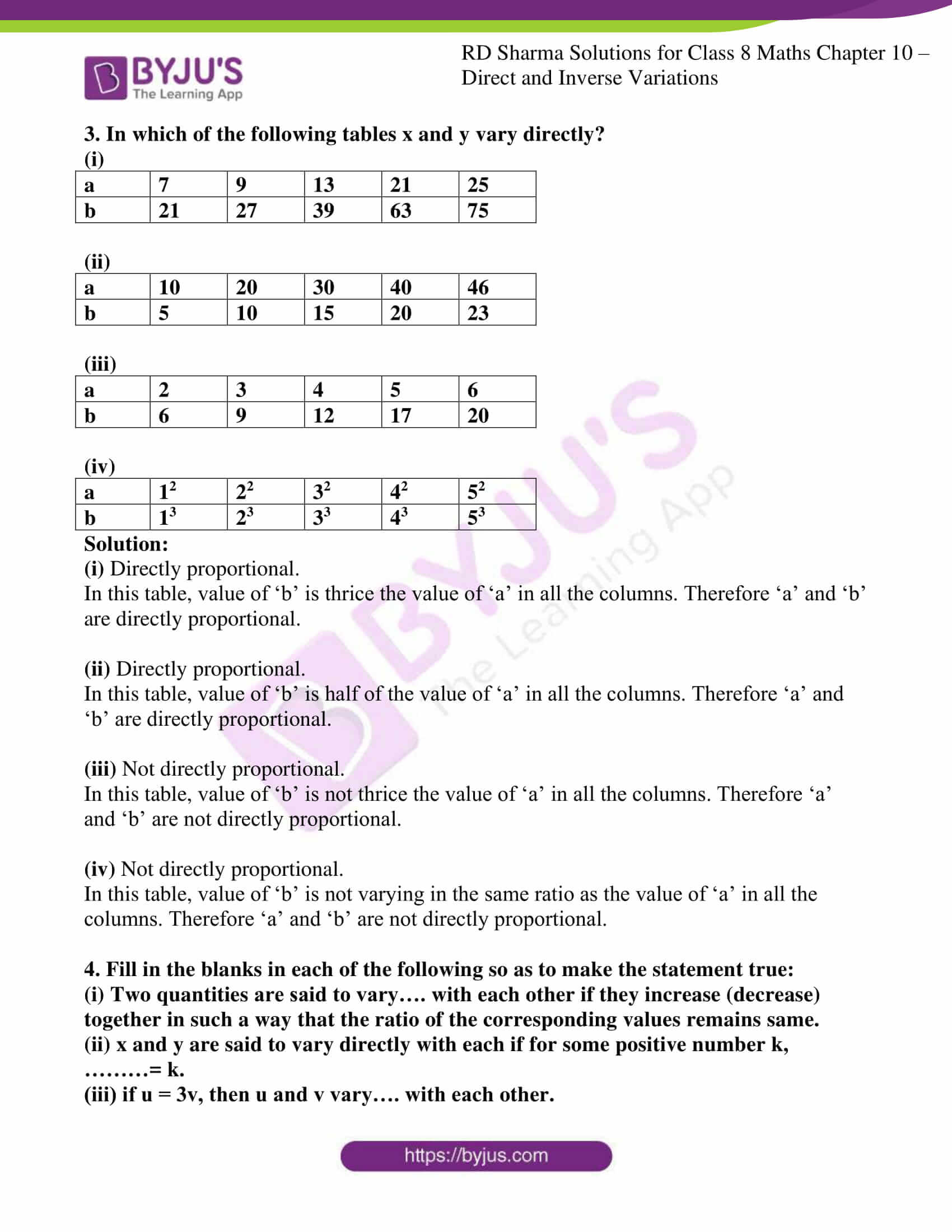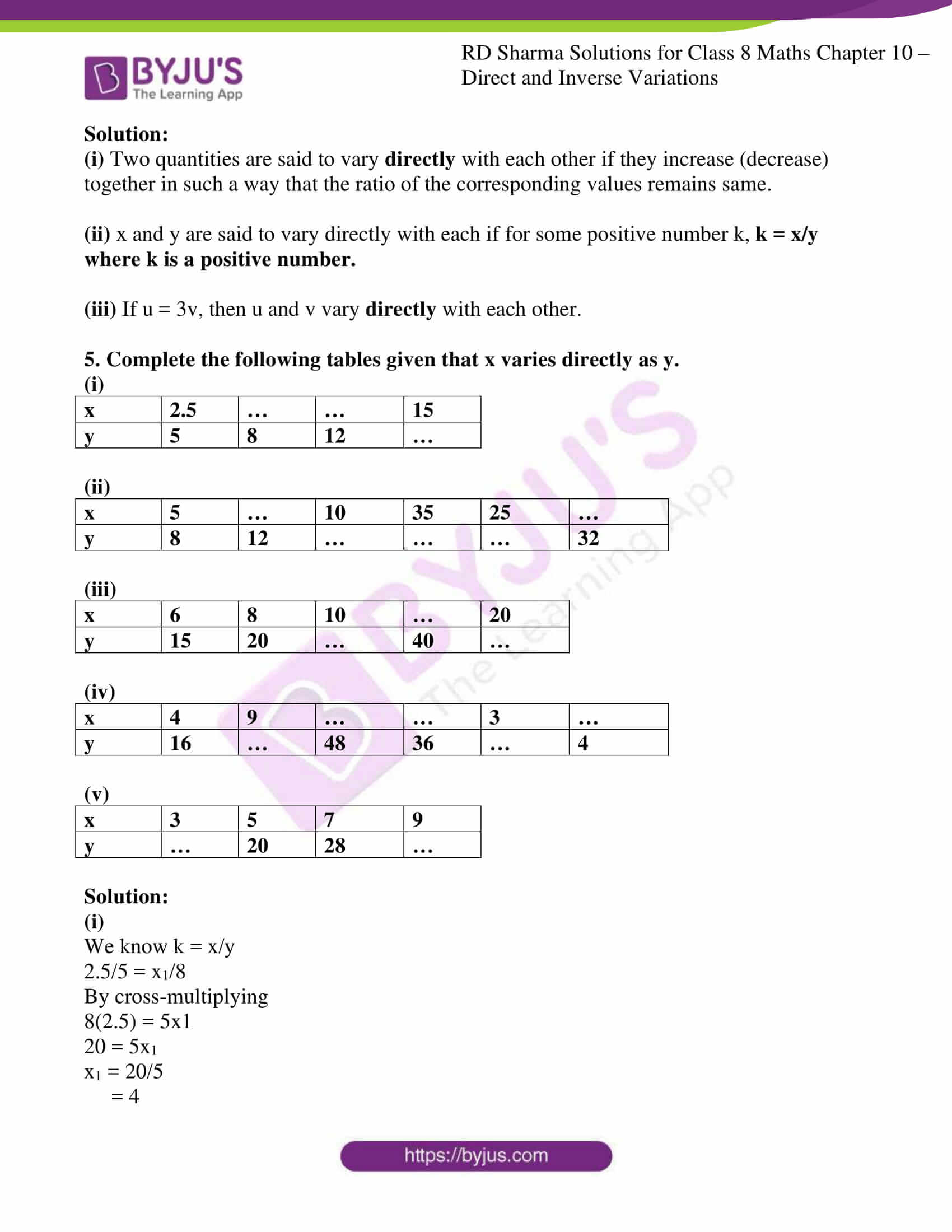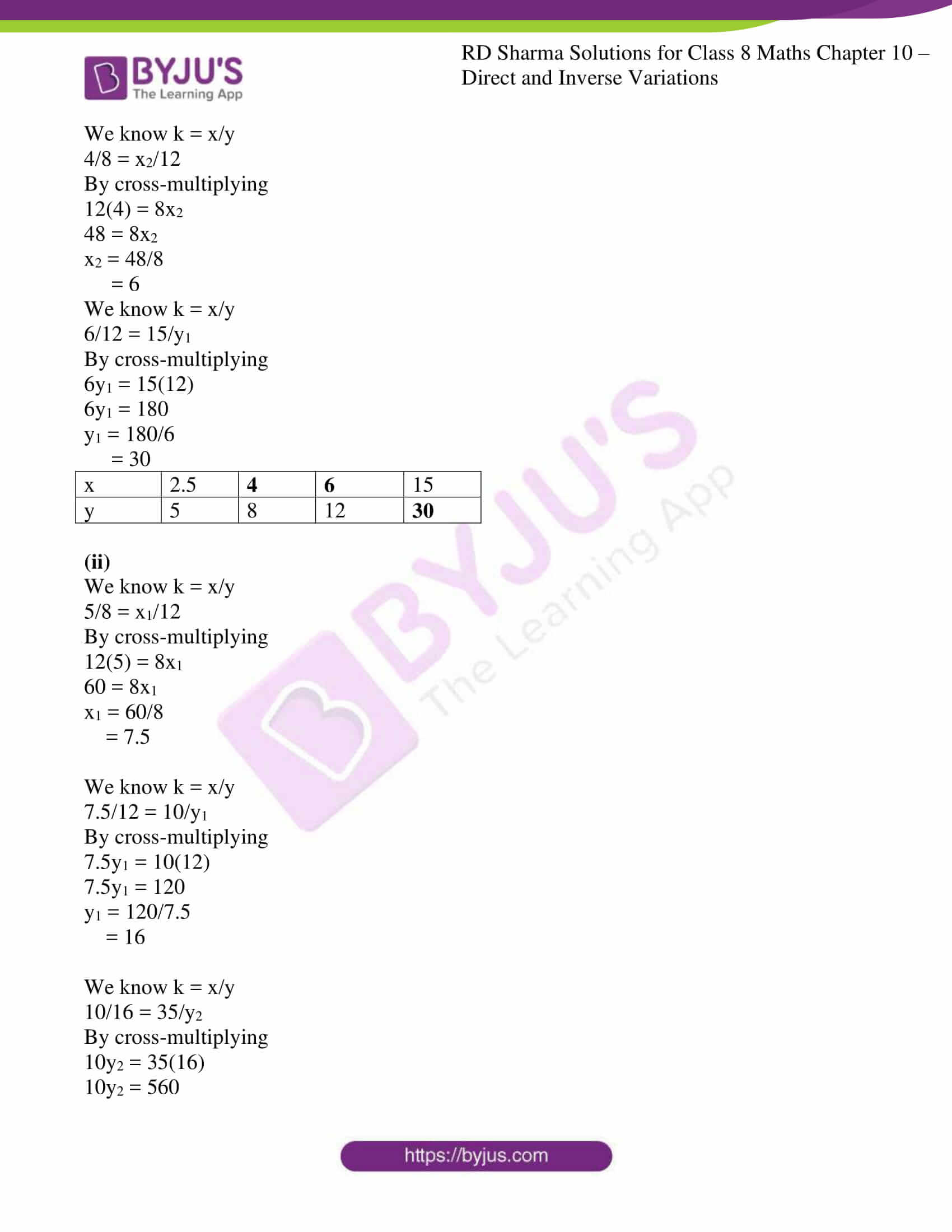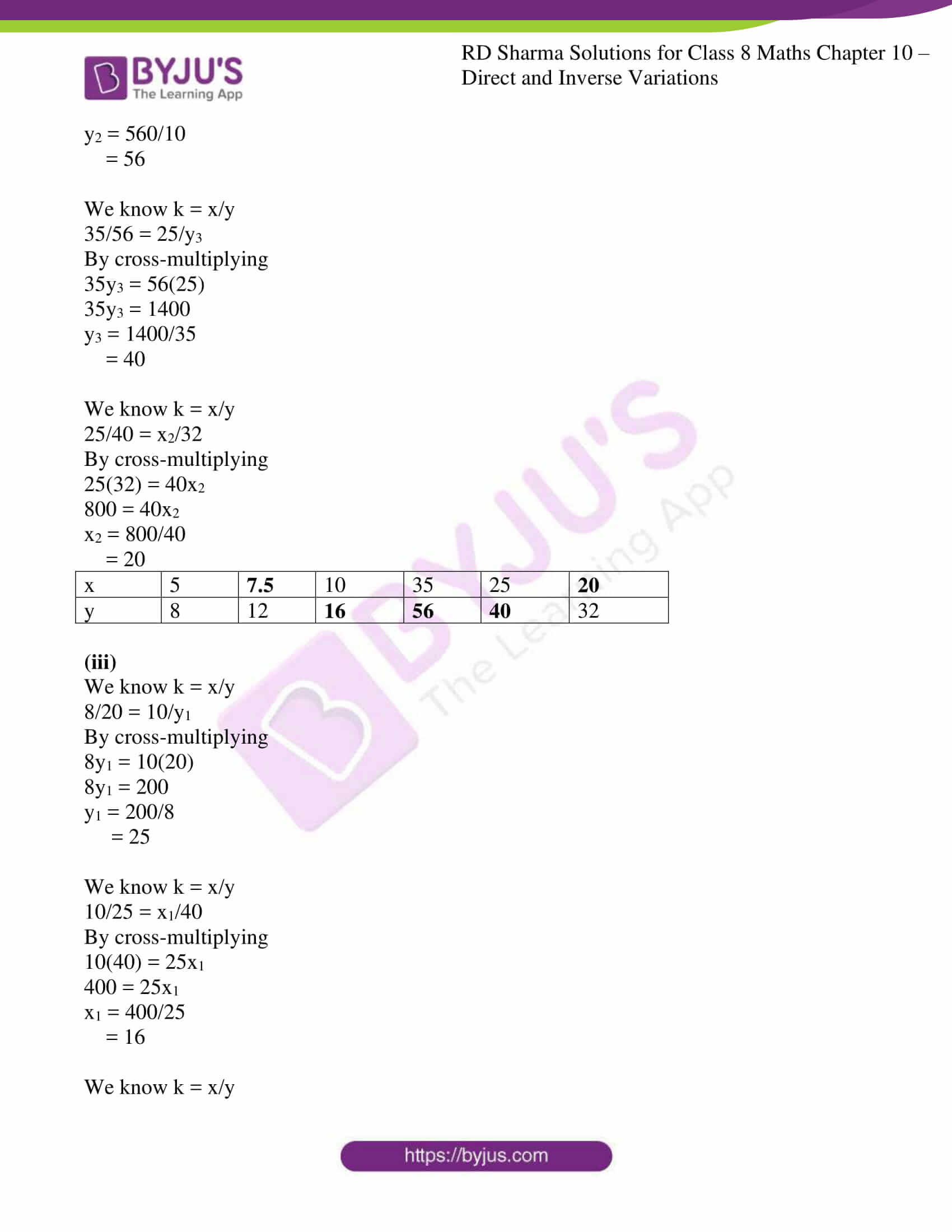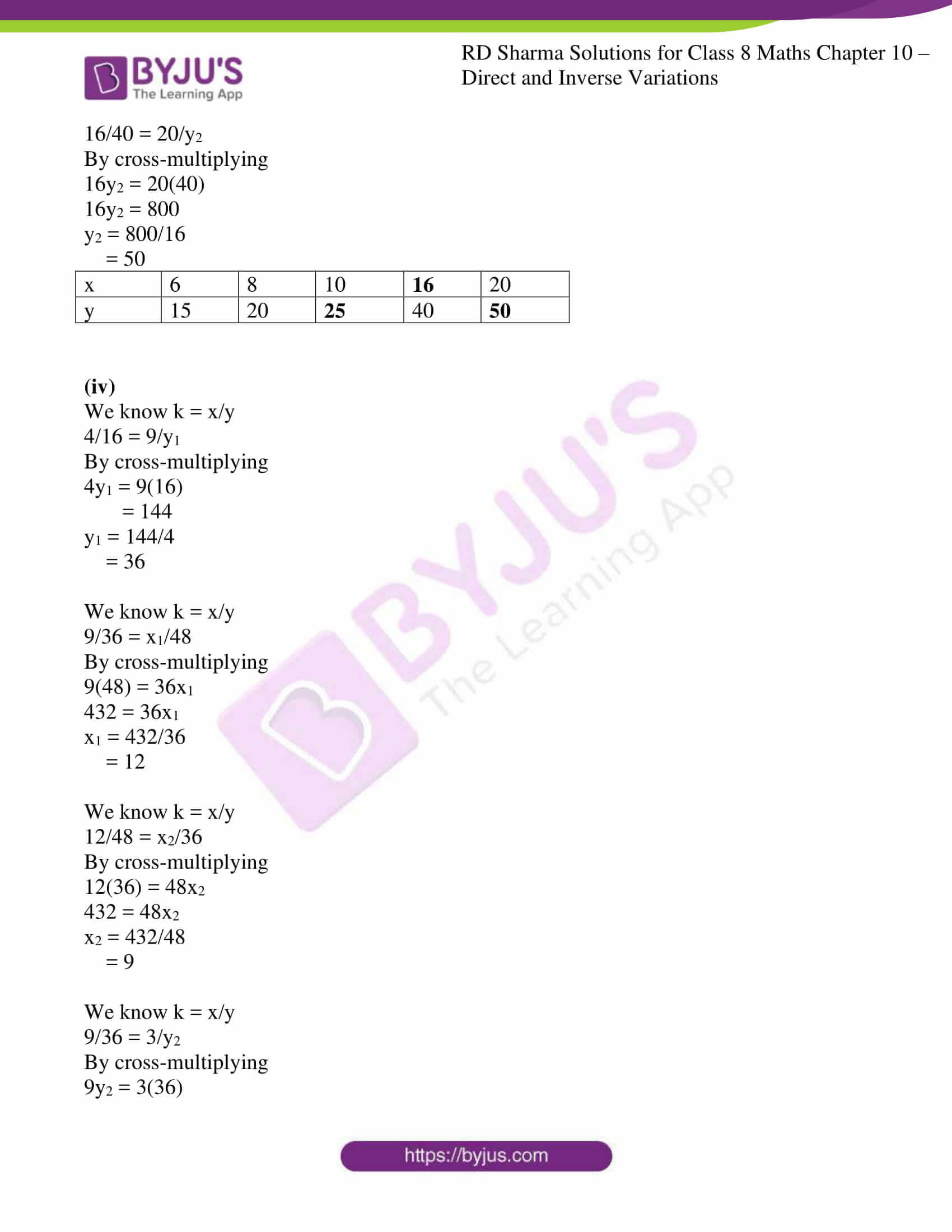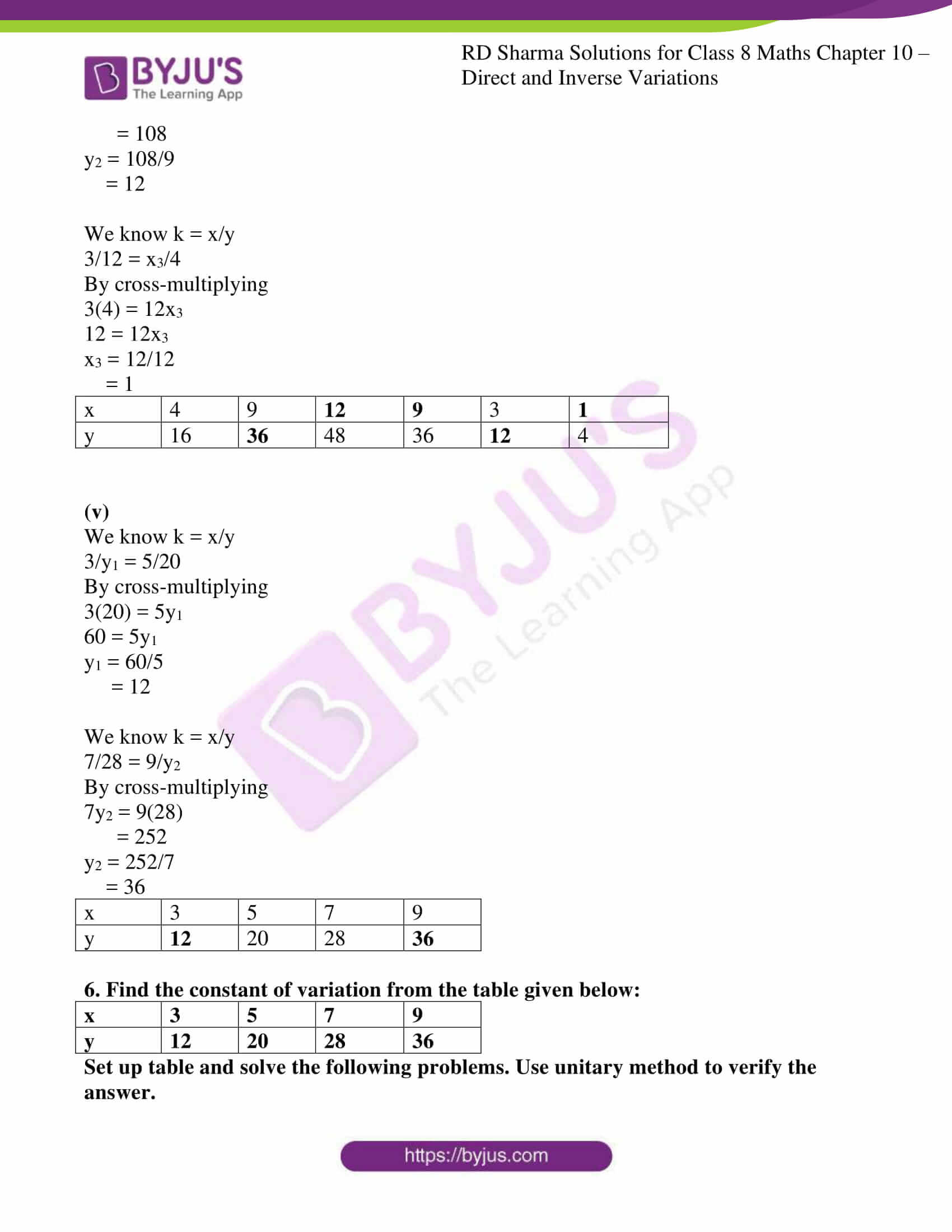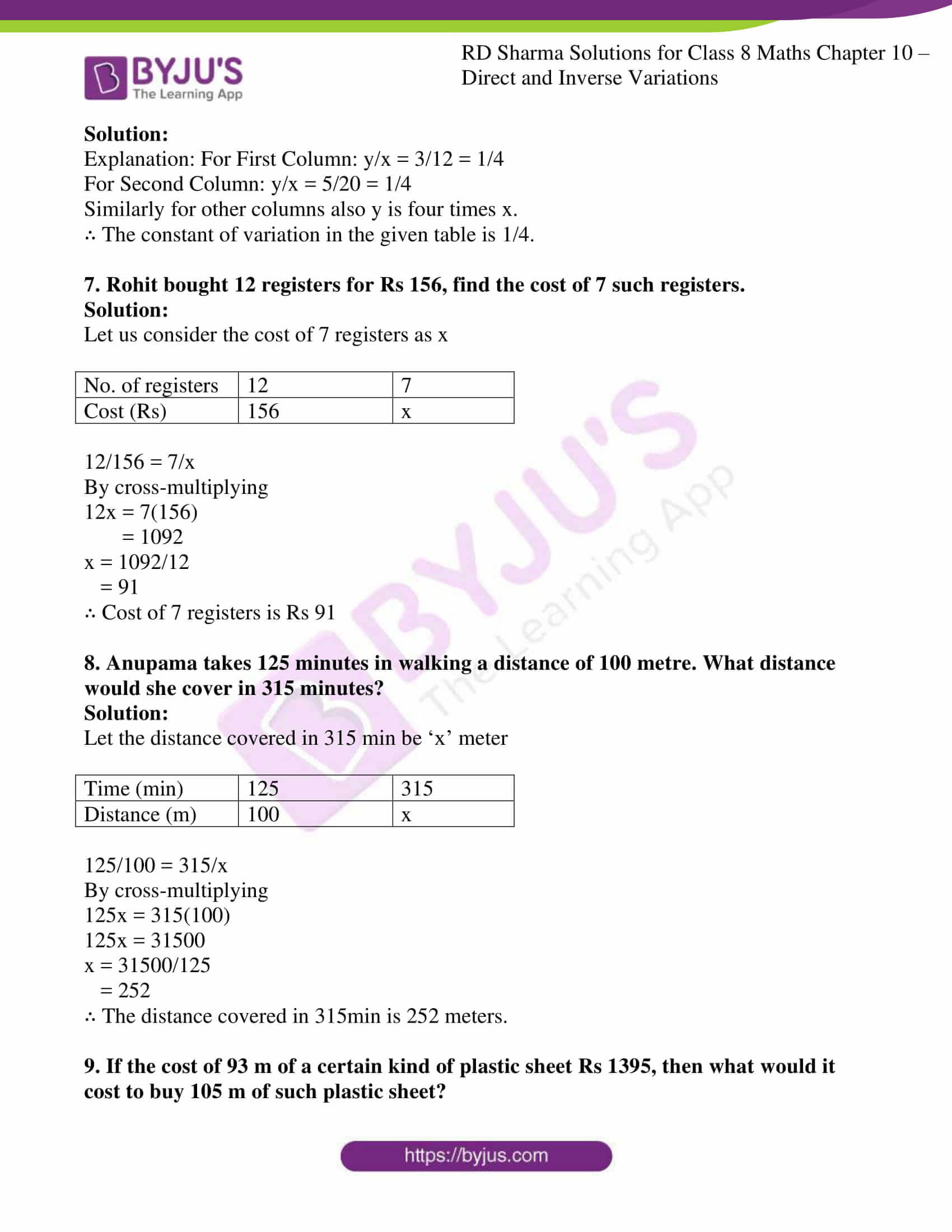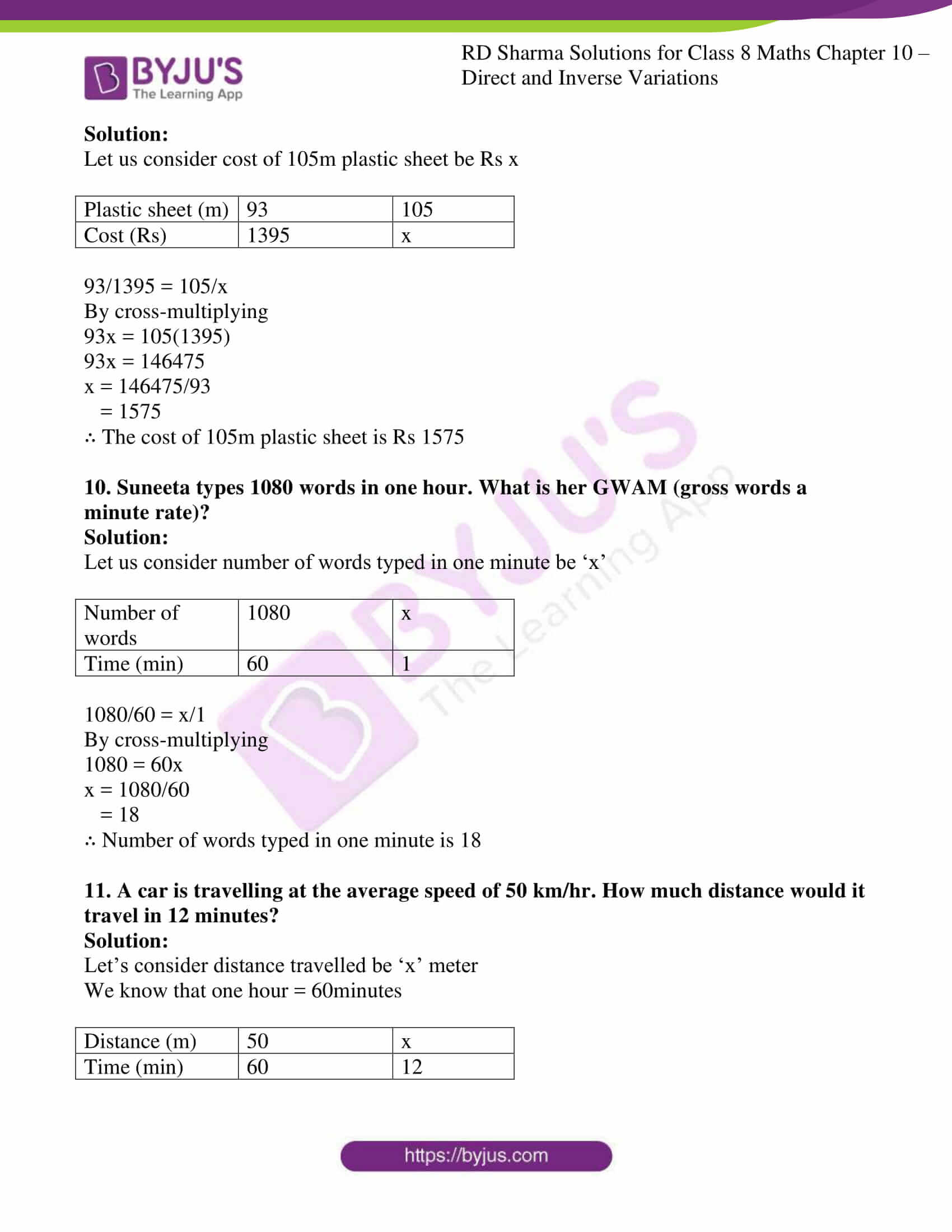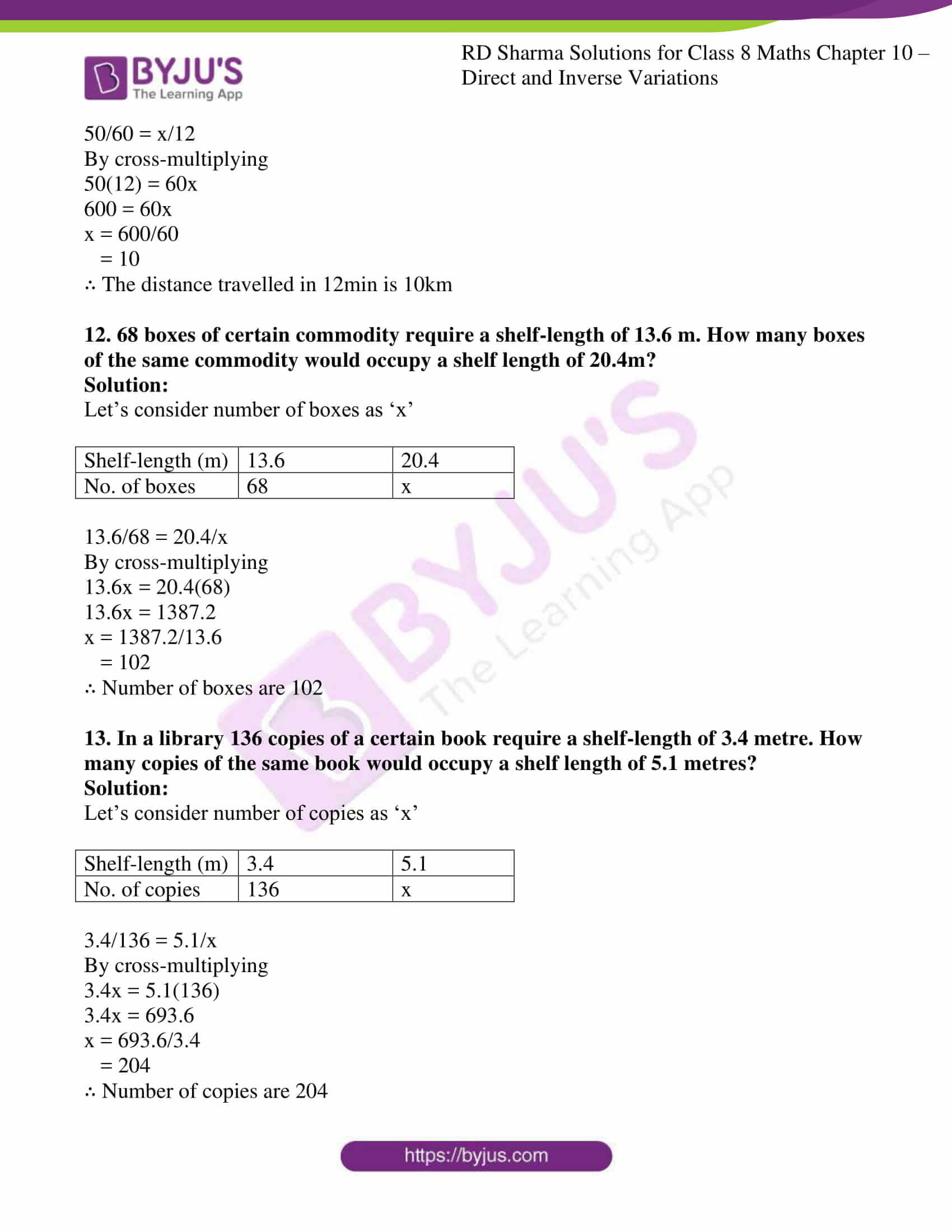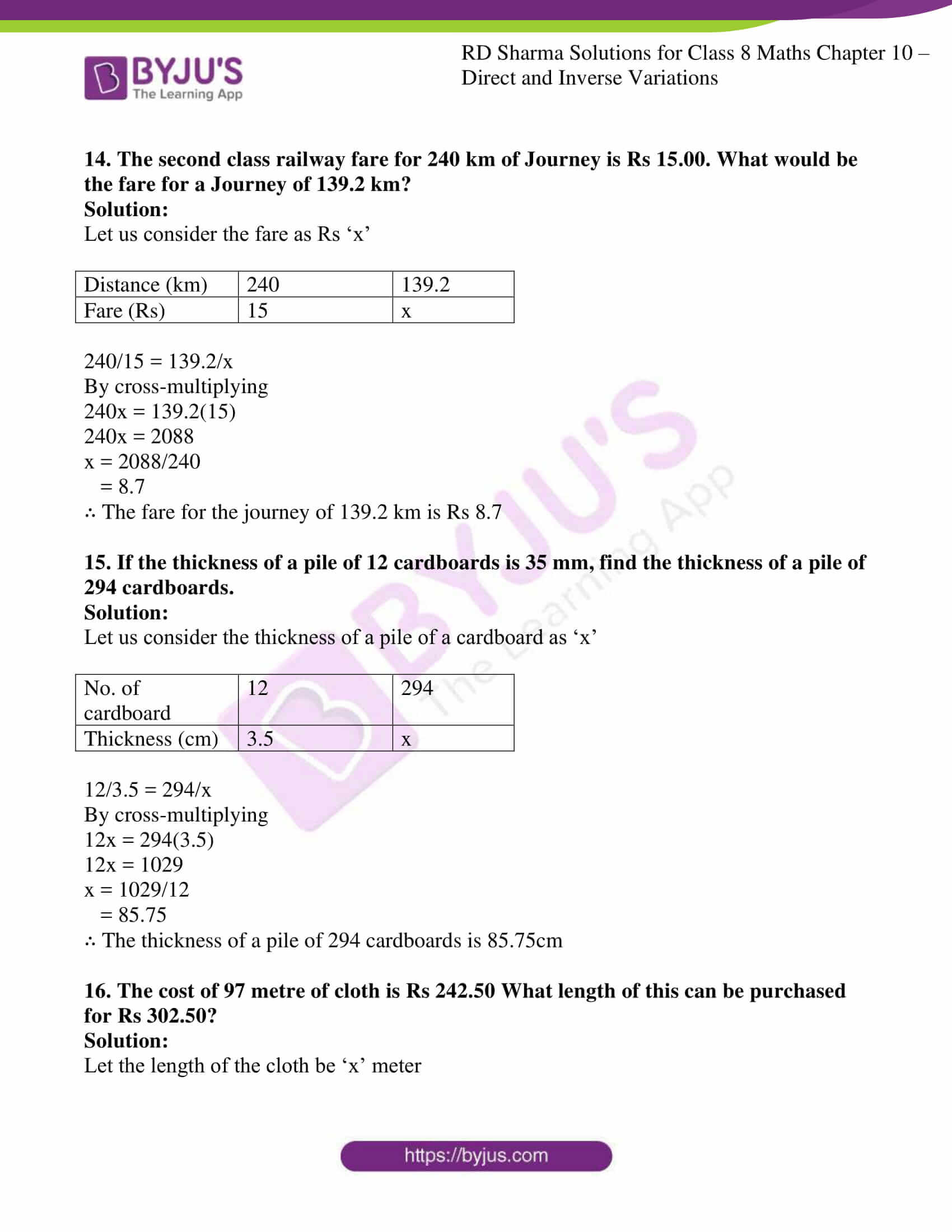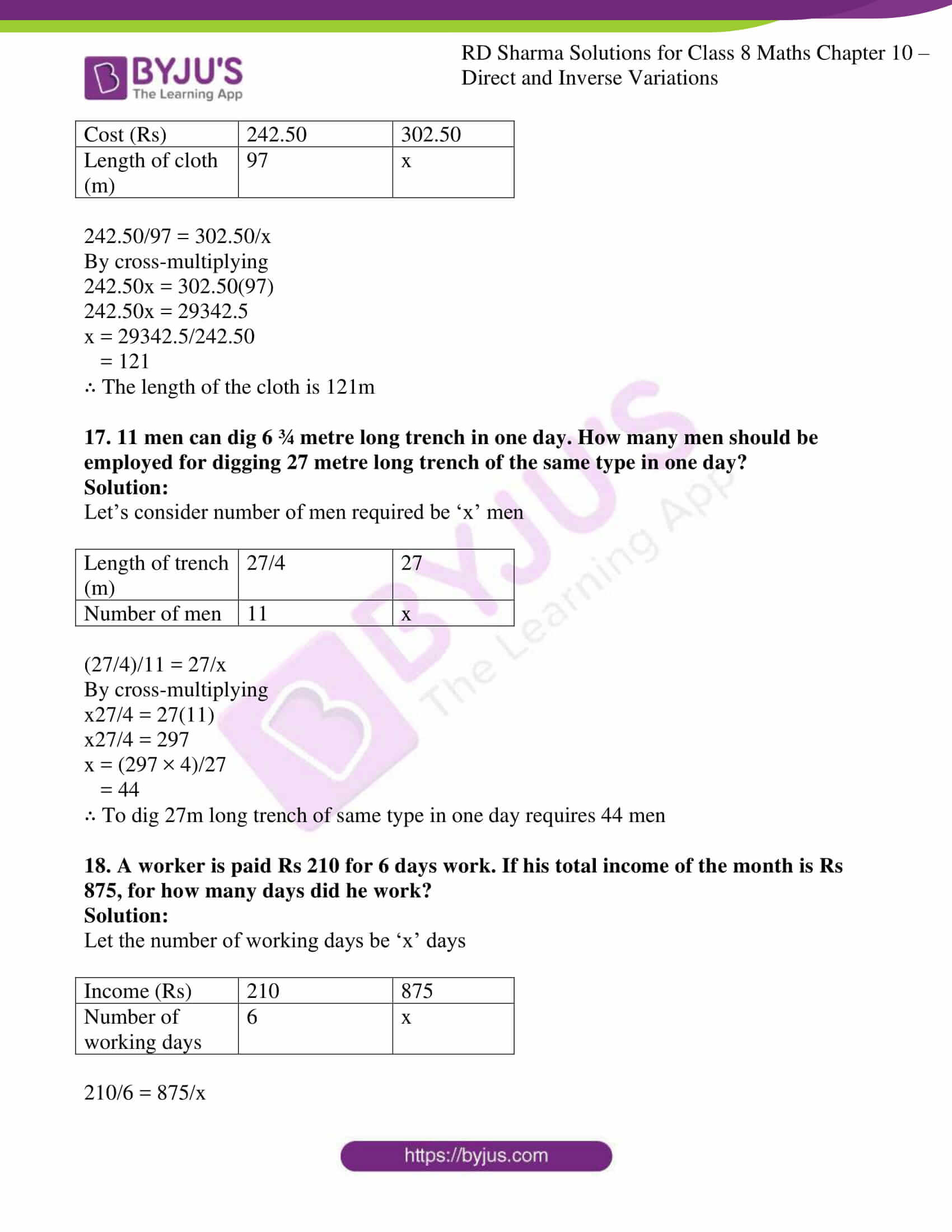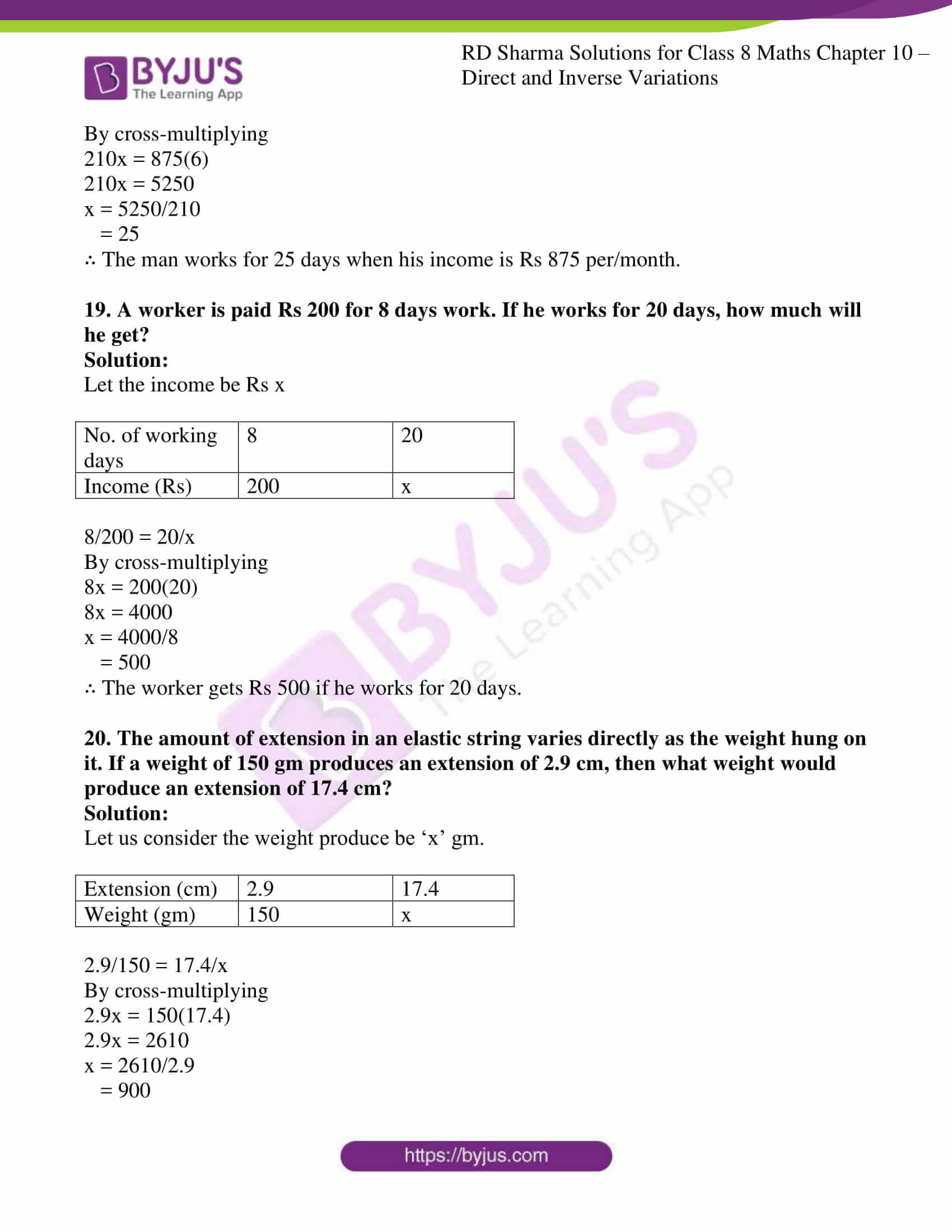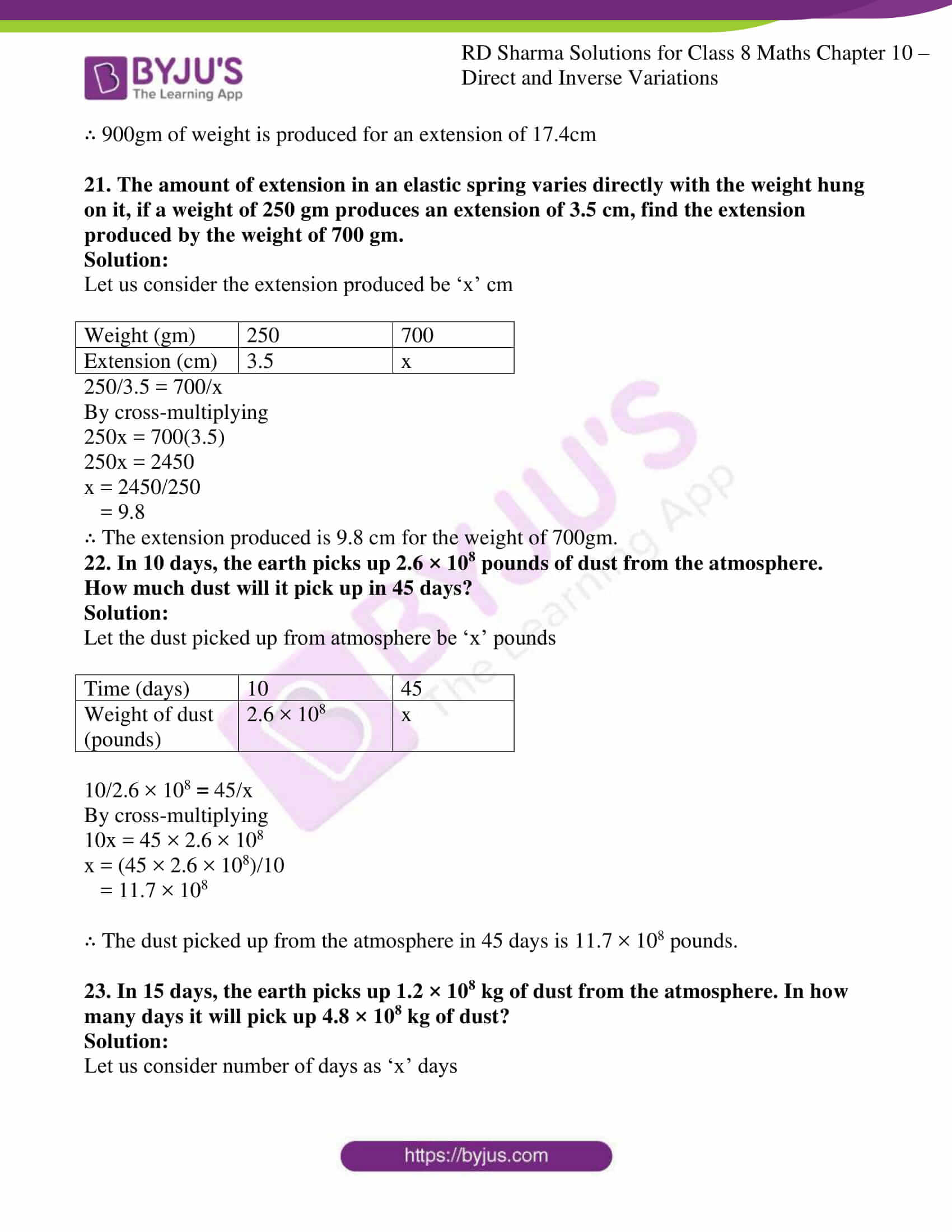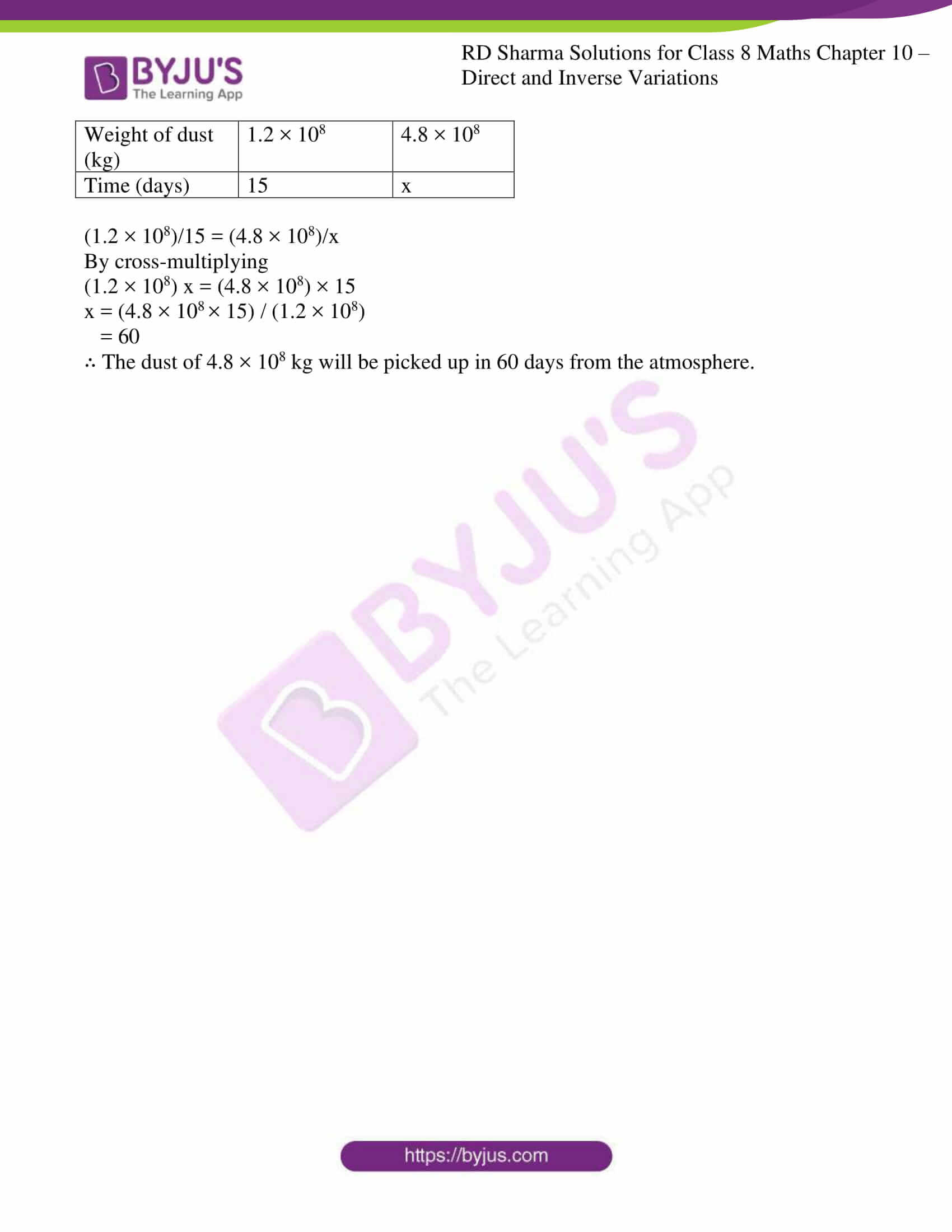### Access Answers to RD Sharma Solutions for Class 8 Maths Exercise 10.1 Chapter 10 Direct and Inverse Variations

1. Explain the concept of direct variation.

Solution:

If the values of two quantities depend on each other in such a way that a change in one quantity results in corresponding change in other, therefore if the ratio between the two variables remains constant, it is said to be in direct variation.

2. Which of the following quantities vary directly with each other?
(i) Number of articles (x) and their price (y).
(ii) Weight of articles (x) and their cost (y).
(iii) Distance x and time y, speed remaining the same.
(iv) Wages (y) and number of hours (x) of work.
(v) Speed (x) and time (y) distance covered remaining the same).
(vi) Area of a land (x) and its cost (y).

Solution:

(i) Number of articles (x) and their price (y)

If number of articles is increasing then cost will also increase. So it is a case of direct proportion

(ii) Weight of articles (x) and their cost (y).

When weight of the article is increasing then cost also increase. So it is a case of direct proportion

(iii) Distance x and time y, speed remaining the same.

Time increases when distance increases, if speed remains constant. So it is a case of direct proportion

(iv) Wages (y) and number of hours (x) of work.

Wages increases if the number of working hours increases. So it is a case of direct proportion

(v) Speed (x) and time (y) distance covered remaining the same).

For same distance time taken will reduce if speed is increased. So it is not a case of direct proportion

(vi) Area of a land (x) and its cost (y).

Cost of the land increases if its area increases. So it is a case of direct proportion

3. In which of the following tables x and y vary directly?

(i)

 a 7 9 13 21 25 b 21 27 39 63 75

(ii)

 a 10 20 30 40 46 b 5 10 15 20 23

(iii)

 a 2 3 4 5 6 b 6 9 12 17 20

(iv)

 a 12 22 32 42 52 b 13 23 33 43 53

Solution:

(i) Directly proportional.

In this table, value of ‘b’ is thrice the value of ‘a’ in all the columns. Therefore ‘a’ and ‘b’ are directly proportional.

(ii) Directly proportional.

In this table, value of ‘b’ is half of the value of ‘a’ in all the columns. Therefore ‘a’ and ‘b’ are directly proportional.

(iii) Not directly proportional.

In this table, value of ‘b’ is not thrice the value of ‘a’ in all the columns. Therefore ‘a’ and ‘b’ are not directly proportional.

(iv) Not directly proportional.

In this table, value of ‘b’ is not varying in the same ratio as the value of ‘a’ in all the columns. Therefore ‘a’ and ‘b’ are not directly proportional.

4. Fill in the blanks in each of the following so as to make the statement true:
(i) Two quantities are said to vary…. with each other if they increase (decrease) together in such a way that the ratio of the corresponding values remains same.
(ii) x and y are said to vary directly with each if for some positive number k, ………= k.

(iii) if u = 3v, then u and v vary…. with each other.

Solution:

(i) Two quantities are said to vary directly with each other if they increase (decrease) together in such a way that the ratio of the corresponding values remains same.

(ii) x and y are said to vary directly with each if for some positive number k, k = x/y where k is a positive number.

(iii) If u = 3v, then u and v vary directly with each other.

5. Complete the following tables given that x varies directly as y.

(i)

 x 2.5 … … 15 y 5 8 12 …

(ii)

 x 5 … 10 35 25 … y 8 12 … … … 32

(iii)

 x 6 8 10 … 20 y 15 20 … 40 …

(iv)

 x 4 9 … … 3 … y 16 … 48 36 … 4

(v)

 x 3 5 7 9 y … 20 28 …

Solution:

(i)

We know k = x/y

2.5/5 = x1/8

By cross-multiplying

8(2.5) = 5×1

20 = 5x1

x1 = 20/5

= 4

We know k = x/y

4/8 = x2/12

By cross-multiplying

12(4) = 8x2

48 = 8x2

x2 = 48/8

= 6

We know k = x/y

6/12 = 15/y1

By cross-multiplying

6y1 = 15(12)

6y1 = 180

y1 = 180/6

= 30

 x 2.5 4 6 15 y 5 8 12 30

(ii)

We know k = x/y

5/8 = x1/12

By cross-multiplying

12(5) = 8x1

60 = 8x1

x1 = 60/8

= 7.5

We know k = x/y

7.5/12 = 10/y1

By cross-multiplying

7.5y1 = 10(12)

7.5y1 = 120

y1 = 120/7.5

= 16

We know k = x/y

10/16 = 35/y2

By cross-multiplying

10y2 = 35(16)

10y2 = 560

y2 = 560/10

= 56

We know k = x/y

35/56 = 25/y3

By cross-multiplying

35y3 = 56(25)

35y3 = 1400

y3 = 1400/35

= 40

We know k = x/y

25/40 = x2/32

By cross-multiplying

25(32) = 40x2

800 = 40x2

x2 = 800/40

= 20

 x 5 7.5 10 35 25 20 y 8 12 16 56 40 32

(iii)

We know k = x/y

8/20 = 10/y1

By cross-multiplying

8y1 = 10(20)

8y1 = 200

y1 = 200/8

= 25

We know k = x/y

10/25 = x1/40

By cross-multiplying

10(40) = 25x1

400 = 25x1

x1 = 400/25

= 16

We know k = x/y

16/40 = 20/y2

By cross-multiplying

16y2 = 20(40)

16y2 = 800

y2 = 800/16

= 50

 x 6 8 10 16 20 y 15 20 25 40 50

(iv)

We know k = x/y

4/16 = 9/y1

By cross-multiplying

4y1 = 9(16)

= 144

y1 = 144/4

= 36

We know k = x/y

9/36 = x1/48

By cross-multiplying

9(48) = 36x1

432 = 36x1

x1 = 432/36

= 12

We know k = x/y

12/48 = x2/36

By cross-multiplying

12(36) = 48x2

432 = 48x2

x2 = 432/48

= 9

We know k = x/y

9/36 = 3/y2

By cross-multiplying

9y2 = 3(36)

= 108

y2 = 108/9

= 12

We know k = x/y

3/12 = x3/4

By cross-multiplying

3(4) = 12x3

12 = 12x3

x3 = 12/12

= 1

 x 4 9 12 9 3 1 y 16 36 48 36 12 4

(v)

We know k = x/y

3/y1 = 5/20

By cross-multiplying

3(20) = 5y1

60 = 5y1

y1 = 60/5

= 12

We know k = x/y

7/28 = 9/y2

By cross-multiplying

7y2 = 9(28)

= 252

y2 = 252/7

= 36

 x 3 5 7 9 y 12 20 28 36

6. Find the constant of variation from the table given below:

 x 3 5 7 9 y 12 20 28 36

Set up table and solve the following problems. Use unitary method to verify the answer.

Solution:

Explanation: For First Column: y/x = 3/12 = 1/4

For Second Column: y/x = 5/20 = 1/4

Similarly for other columns also y is four times x.

∴ The constant of variation in the given table is 1/4.

7. Rohit bought 12 registers for Rs 156, find the cost of 7 such registers.

Solution:

Let us consider the cost of 7 registers as x

 No. of registers 12 7 Cost (Rs) 156 x

12/156 = 7/x

By cross-multiplying

12x = 7(156)

= 1092

x = 1092/12

= 91

∴ Cost of 7 registers is Rs 91

8. Anupama takes 125 minutes in walking a distance of 100 metre. What distance would she cover in 315 minutes?

Solution:

Let the distance covered in 315 min be ‘x’ meter

 Time (min) 125 315 Distance (m) 100 x

125/100 = 315/x

By cross-multiplying

125x = 315(100)

125x = 31500

x = 31500/125

= 252

∴ The distance covered in 315min is 252 meters.

9. If the cost of 93 m of a certain kind of plastic sheet Rs 1395, then what would it cost to buy 105 m of such plastic sheet?

Solution:

Let us consider cost of 105m plastic sheet be Rs x

 Plastic sheet (m) 93 105 Cost (Rs) 1395 x

93/1395 = 105/x

By cross-multiplying

93x = 105(1395)

93x = 146475

x = 146475/93

= 1575

∴ The cost of 105m plastic sheet is Rs 1575

10. Suneeta types 1080 words in one hour. What is her GWAM (gross words a minute rate)?

Solution:

Let us consider number of words typed in one minute be ‘x’

 Number of words 1080 x Time (min) 60 1

1080/60 = x/1

By cross-multiplying

1080 = 60x

x = 1080/60

= 18

∴ Number of words typed in one minute is 18

11. A car is travelling at the average speed of 50 km/hr. How much distance would it travel in 12 minutes?

Solution:

Let’s consider distance travelled be ‘x’ meter

We know that one hour = 60minutes

 Distance (m) 50 x Time (min) 60 12

50/60 = x/12

By cross-multiplying

50(12) = 60x

600 = 60x

x = 600/60

= 10

∴ The distance travelled in 12min is 10km

12. 68 boxes of certain commodity require a shelf-length of 13.6 m. How many boxes of the same commodity would occupy a shelf length of 20.4m?

Solution:

Let’s consider number of boxes as ‘x’

 Shelf-length (m) 13.6 20.4 No. of boxes 68 x

13.6/68 = 20.4/x

By cross-multiplying

13.6x = 20.4(68)

13.6x = 1387.2

x = 1387.2/13.6

= 102

∴ Number of boxes are 102

13. In a library 136 copies of a certain book require a shelf-length of 3.4 metre. How many copies of the same book would occupy a shelf length of 5.1 metres?

Solution:

Let’s consider number of copies as ‘x’

 Shelf-length (m) 3.4 5.1 No. of copies 136 x

3.4/136 = 5.1/x

By cross-multiplying

3.4x = 5.1(136)

3.4x = 693.6

x = 693.6/3.4

= 204

∴ Number of copies are 204

14. The second class railway fare for 240 km of Journey is Rs 15.00. What would be the fare for a Journey of 139.2 km?

Solution:

Let us consider the fare as Rs ‘x’

 Distance (km) 240 139.2 Fare (Rs) 15 x

240/15 = 139.2/x

By cross-multiplying

240x = 139.2(15)

240x = 2088

x = 2088/240

= 8.7

∴ The fare for the journey of 139.2 km is Rs 8.7

15. If the thickness of a pile of 12 cardboards is 35 mm, find the thickness of a pile of 294 cardboards.

Solution:

Let us consider the thickness of a pile of a cardboard as ‘x’

 No. of cardboard 12 294 Thickness (cm) 3.5 x

12/3.5 = 294/x

By cross-multiplying

12x = 294(3.5)

12x = 1029

x = 1029/12

= 85.75

∴ The thickness of a pile of 294 cardboards is 85.75cm

16. The cost of 97 metre of cloth is Rs 242.50 What length of this can be purchased for Rs 302.50?

Solution:

Let the length of the cloth be ‘x’ meter

 Cost (Rs) 242.5 302.50 Length of cloth (m) 97 x

242.50/97 = 302.50/x

By cross-multiplying

242.50x = 302.50(97)

242.50x = 29342.5

x = 29342.5/242.50

= 121

∴ The length of the cloth is 121m

17. 11 men can dig 6 ¾ metre long trench in one day. How many men should be employed for digging 27 metre long trench of the same type in one day?

Solution:

Let’s consider number of men required be ‘x’ men

 Length of trench (m) 27/4 27 Number of men 11 x

(27/4)/11 = 27/x

By cross-multiplying

x27/4 = 27(11)

x27/4 = 297

x = (297 × 4)/27

= 44

∴ To dig 27m long trench of same type in one day requires 44 men

18. A worker is paid Rs 210 for 6 days work. If his total income of the month is Rs 875, for how many days did he work?

Solution:

Let the number of working days be ‘x’ days

 Income (Rs) 210 875 Number of working days 6 x

210/6 = 875/x

By cross-multiplying

210x = 875(6)

210x = 5250

x = 5250/210

= 25

∴ The man works for 25 days when his income is Rs 875 per/month.

19. A worker is paid Rs 200 for 8 days work. If he works for 20 days, how much will he get?

Solution:

Let the income be Rs x

 No. of working days 8 20 Income (Rs) 200 x

8/200 = 20/x

By cross-multiplying

8x = 200(20)

8x = 4000

x = 4000/8

= 500

∴ The worker gets Rs 500 if he works for 20 days.

20. The amount of extension in an elastic string varies directly as the weight hung on it. If a weight of 150 gm produces an extension of 2.9 cm, then what weight would produce an extension of 17.4 cm?

Solution:

Let us consider the weight produce be ‘x’ gm.

 Extension (cm) 2.9 17.4 Weight (gm) 150 x

2.9/150 = 17.4/x

By cross-multiplying

2.9x = 150(17.4)

2.9x = 2610

x = 2610/2.9

= 900

∴ 900gm of weight is produced for an extension of 17.4cm

21. The amount of extension in an elastic spring varies directly with the weight hung on it, if a weight of 250 gm produces an extension of 3.5 cm, find the extension produced by the weight of 700 gm.

Solution:

Let us consider the extension produced be ‘x’ cm

 Weight (gm) 250 700 Extension (cm) 3.5 x

250/3.5 = 700/x

By cross-multiplying

250x = 700(3.5)

250x = 2450

x = 2450/250

= 9.8

∴ The extension produced is 9.8 cm for the weight of 700gm.

22. In 10 days, the earth picks up 2.6 × 108 pounds of dust from the atmosphere. How much dust will it pick up in 45 days?

Solution:

Let the dust picked up from atmosphere be ‘x’ pounds

 Time (days) 10 45 Weight of dust (pounds) 2.6 × 108 x

10/2.6 × 108 = 45/x

By cross-multiplying

10x = 45 × 2.6 × 108

x = (45 × 2.6 × 108)/10

= 11.7 × 108

∴ The dust picked up from the atmosphere in 45 days is 11.7 × 108 pounds.

23. In 15 days, the earth picks up 1.2 × 108 kg of dust from the atmosphere. In how many days it will pick up 4.8 × 108 kg of dust?

Solution:

Let us consider number of days as ‘x’ days

 Weight of dust (kg) 1.2 × 108 4.8 × 108 Time (days) 15 x

(1.2 × 108)/15 = (4.8 × 108)/x

By cross-multiplying

(1.2 × 108) x = (4.8 × 108) × 15

x = (4.8 × 108 × 15) / (1.2 × 108)

= 60

∴ The dust of 4.8 × 108 kg will be picked up in 60 days from the atmosphere.